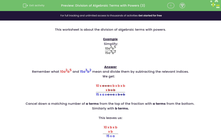# Divide Complicated Algebraic Terms with Powers

In this worksheet, students will divide algebraic terms giving their answers as a single fraction.Key stage:  KS 3

Curriculum topic:   Algebra

Curriculum subtopic:   Use and Interpret Algebraic Notation

Difficulty level:#### Worksheet Overview

This activity is about the division of algebraic terms with powers.

Example

Simplify:

 10a2b5 15a3b2

Remember what 10a2b5 and 15a3b2 mean and divide them by subtracting the relevant indices.

We get:

 10 x a x a x b x b x b x b x b 15 x a x a x a x b x b

Cancel down a matching number of a terms from the top of the fraction with a terms from the bottom.  Similarly with b terms.

This leaves us with:

 10 x b x b x b 15 x a

which reduces by dividing top and bottom by 5 to

 2b3 3a

However, we need not write out our method like this each time.

Remember the rules of indices for division:

a÷ an = am-n

In the example above, we had b5 ÷ b2 = b5 - 2 = b3.

Let's try some questions now.

### What is EdPlace?

We're your National Curriculum aligned online education content provider helping each child succeed in English, maths and science from year 1 to GCSE. With an EdPlace account you’ll be able to track and measure progress, helping each child achieve their best. We build confidence and attainment by personalising each child’s learning at a level that suits them.

Get started